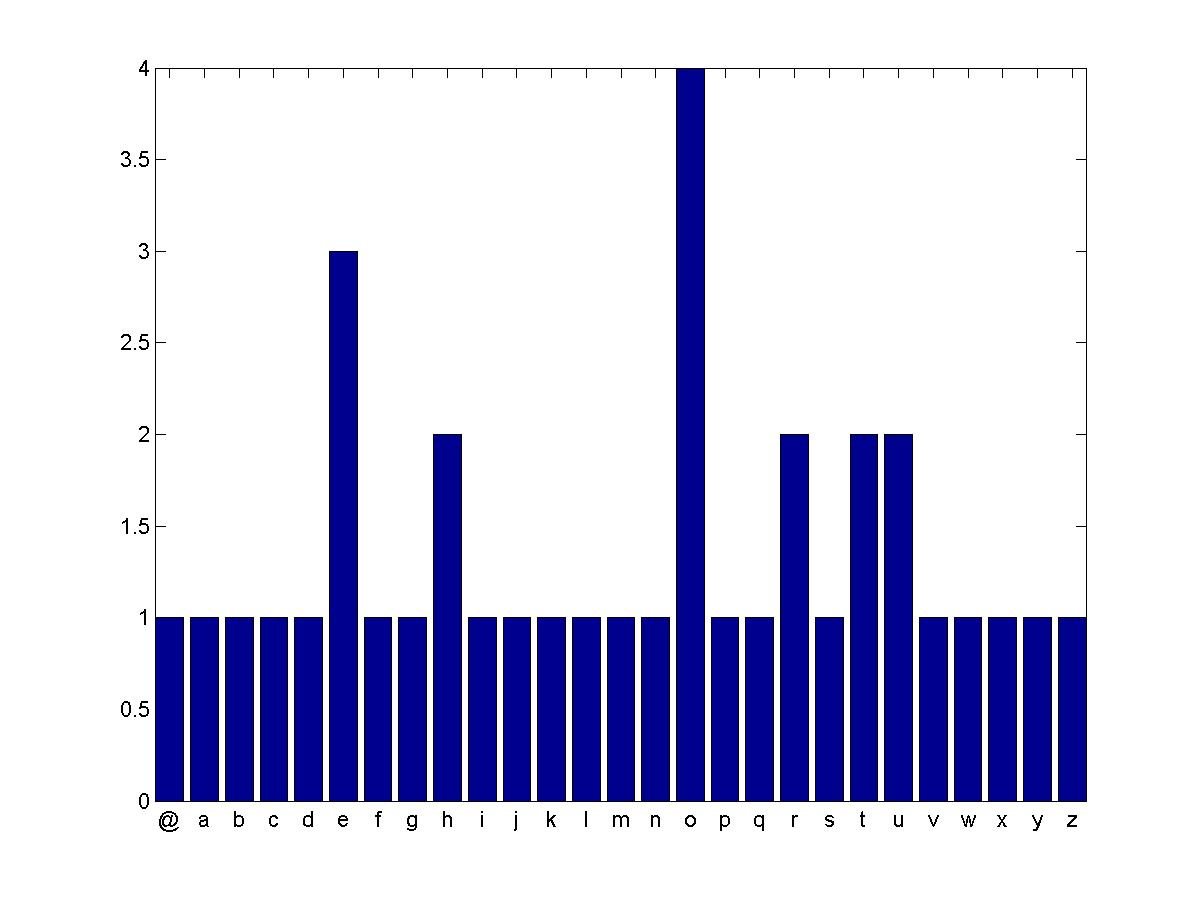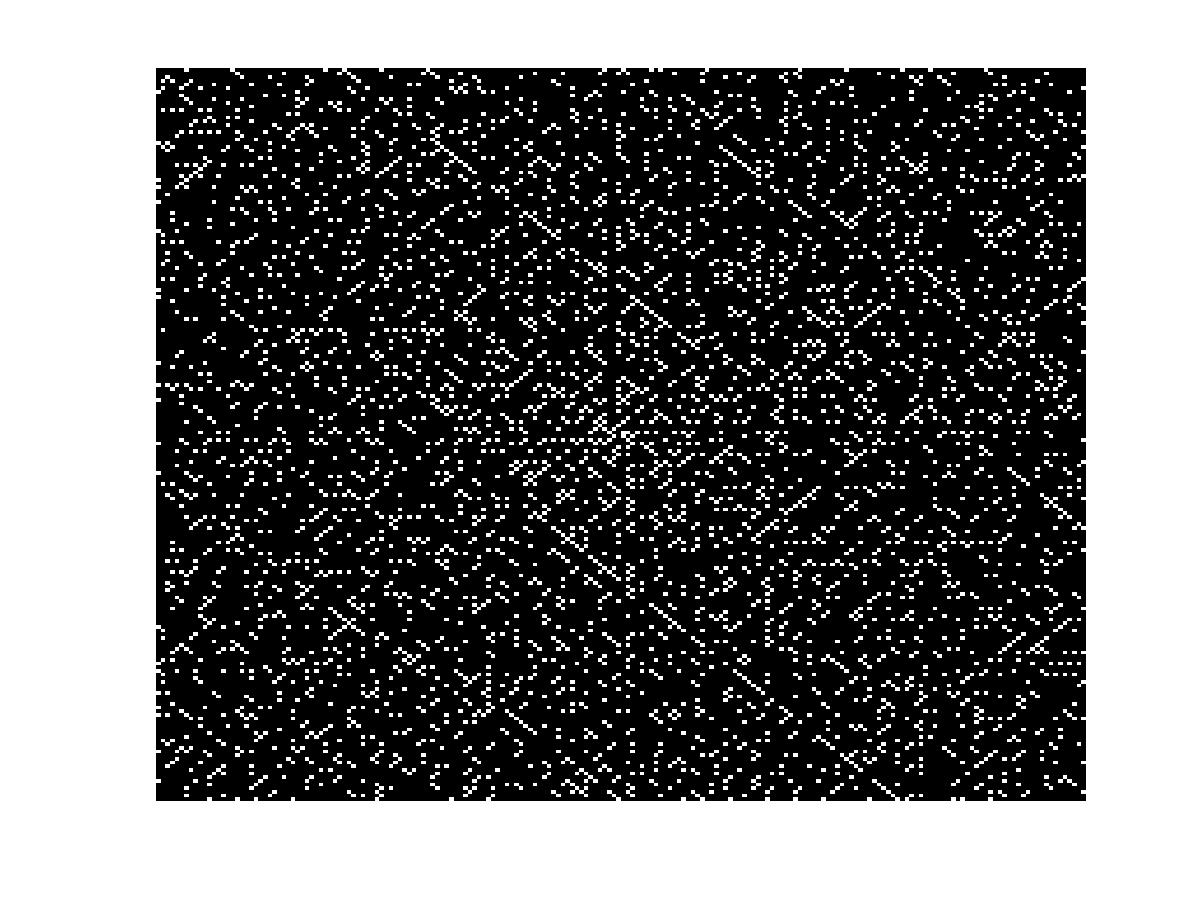## Loren on the Art of MATLABTurn ideas into MATLAB

Note

Loren on the Art of MATLAB has been retired and will not be updated.

# Strings and Numbers as Arguments to Standalone Executables

Guest blogger Peter Webb returns with another in an occasional series of postings about application deployment.

### Contents

Most MATLAB functions take input arguments. When you create a shared library or DLL from a MATLAB function with MATLAB Compiler, the generated library functions take the same number and type of arguments as the MATLAB functions. But what about a standalone executable? How do you pass arguments to a MATLAB function that you've compiled into a complete program?

On the UNIX or DOS command line, of course.

The main program generated by MATLAB Compiler examines the command line and passes any arguments to your main function, the function you listed first when you created the application with mcc. But UNIX and DOS don't understand MATLAB data types, so all they can do is pass the command line arguments to your program as strings.

For example, you might compile the function compute into a standalone executable and then run it with the argument 17:

 compute 17

Your compute function receives the string '17' as its first input. If it is expecting a number, compute probably won't produce the correct result when invoked with a string.

All is not lost, however. Simple modifications to your M-code can enable you to handle many different types of arguments. Let's begin by looking at techniques for handling strings.

### String Arguments

Since MATLAB Compiler generates code that passes strings on the command line to your program's main function, you don't need to modify programs that accept string inputs. For example, here's a program that plots a histogram of the number of times each letter in the alphabet appears in an input string. The function takes a single input and has zero outputs.

function freq(msg)
% Remove spaces
msg(msg == ' ') = [];
  % Convert to lower case, and map the letters to numbers.
% a=1, b=2, etc.
msg = lower(msg) - double('a') + 1;
  % Map non-letter characters to zero.
msg(msg < 1) = 0;
msg(msg > 26) = 0;
 % Display a histogram of the letter frequency in the message.
count = hist(msg, 27);
bar(0:26, count);
labels = char([double('@'), double('a'):double('z')])';
set(gca, 'XTick', 0:26+0.5, 'XTickLabel', labels);
axis tight

First, compile the program to a standalone executable:

mcc -mv freq

Run it with a string argument:

freq "The quick brown fox jumps over the lazy dog!"

The histogram is surprisingly uniform.### Numeric Arguments

The Ulam spiral reveals patterns in the distribution of prime numbers. The MATLAB function ulam below, displays an Ulam spiral as an NxN black and white image.

function ulam(n)
% Compute the NxN ulam spiral
u = spiral(n);
  % Set p(i,j) to 1 iff u(i,j) is prime.
p = isprime(u);
  % Display the results: primes as white pixels
image(p);
colormap([0 0 0; 1 1 1]);
axis off

Create a standalone executable ulam with MATLAB Compiler:

mcc -mv ulam.m

Run the executable to display a 200x200 Ulam spiral:

ulam 200

Not so fast! ulam reports an error:

??? Error using ==> zeros
Trailing string input must be a valid numeric class name.
Error in ==> spiral at 9

If I modify the ulam MATLAB function to expect a string, by calling str2double on the input n, calling ulam in MATLAB will be awkward:

 ulam('200');

Therefore, ulam needs additional logic when it is running as a deployed application. There's a function for that -- isdeployed -- which returns true only when run in a deployed application. I can test for deployed mode, and then convert n from a string into a double, like so:

  if isdeployed
n = str2double(n);
end

Alternatively, I could test to see if n is a character string using ischar:

  if ischar(n)
n = str2double(n);
end

Add either of these tests as the first three lines of the ulam function, and recompile. Now ulam 200 generates an intriguing image. (Why do the primes seem to line up on the diagonals?)In a later posting, I'll demonstrate how to pass cell arrays and other MATLAB data types to your standalone applications. In the meantime, if you have questions or comments, let me know here.

Published with MATLAB® 7.11

|